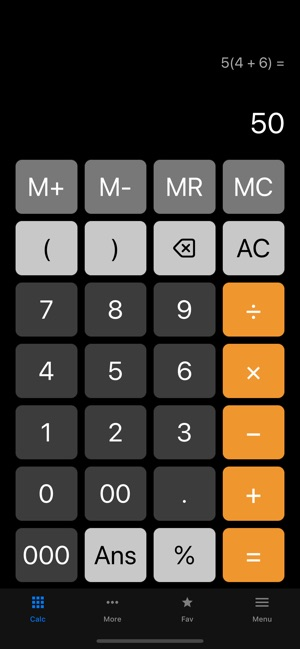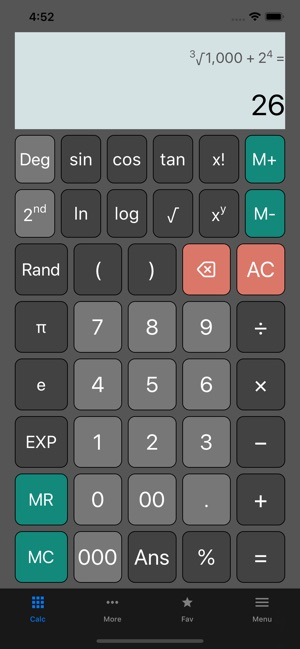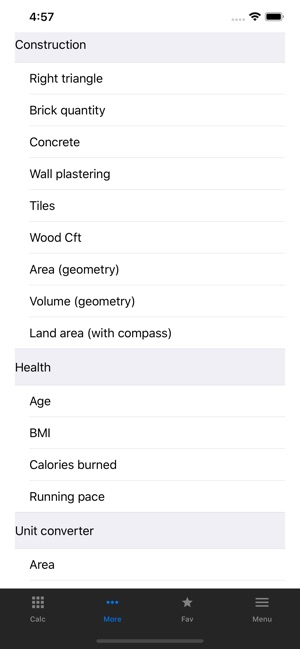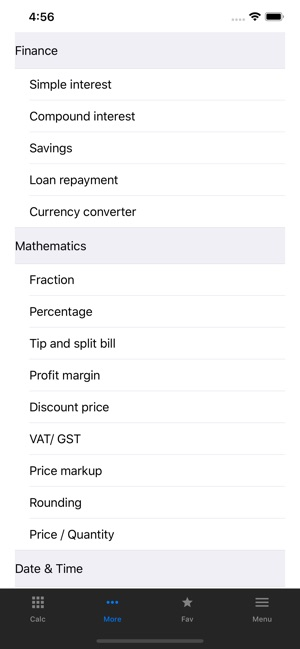Screenshots

••••Description

All-in-one Calculator is a free calculator app for iPhone and iPad featuring over 40+ calculators and unit converters categorized under finance, health, date & time, construction, shopping and everyday math.

Simple yet powerful, the following features are built into this all-in-one calculator app:-

STANDARD CALCULATOR
• Includes percent key, negative sign, memory functions, brackets and history viewer.
• Advanced mode includes functions for trigonometry, roots, exponents and logarithm found in scientific calculators.

UNIT CONVERTER
• Convert values between metric/ imperial units with conversion calculators for temperature, length, weight, area, volume and many more.

FINANCIAL CALCULATORS
• Interest calculators using simple and compound interest
• Loan calculator with amortization schedule
• Savings calculator to calculate future value and monthly savings amount
• Currency converter

HEALTH
-Keep track of your body weight with these calculators:-
• BMI calculator
• Calories burnt calculator
• Running Pace calculator

EVERYDAY MATH
• Percentage calculator
• Fraction calculator
• Rounding calculator
• Area/ Volume calculator
• Right-triangle calculator

CONSTRUCTION
-Estimate material requirements for small repair works/ DIY projects with these calculators:-
• Brick calculator
• Concrete calculator
• Tiles calculator
• Lumber volume calculator
• Land area calculator

DATE & TIME
-Find duration between two date/ time values in months, weeks, days, hours, and minutes.
• Date calculator (Also useful for age calculation)
• Time calculator
• Calculators to add/ subtract date and time

-These calculators are useful when shopping and for those who run small business.
• Profit margin calculator
• Price markup calculator
• Discount calculator
• Tip and split calculator
• Sales tax/ VAT calculator

What’s New

Version 1.2.6

Code migration to Swift 5
Bug fixes and minor improvements

5.0 out of 5
3 Ratings

3 Ratings

Information

Provider
tasser gyati
Size
26.8 MB
Category
Productivity
Compatibility

Requires iOS 10.0 or later. Compatible with iPhone, iPad and iPod touch.

Languages

English, French, German, Italian, Japanese, Korean, Portuguese, Russian, Simplified Chinese, Spanish, Traditional Chinese

Age Rating
Rated 4+
Price
Free

Supports

•